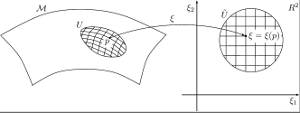# Information geometry and machine learning

 Title: Information geometry and machine learningImage:manifold.jpg Description: In machine learning, we often introduce probabilistic models to handle uncertainty in the data, and most of the times due to the computational cost, we end up selecting (a priori, or even at run time) a subset of all possible statistical models for the variables that appear in the problem. From a geometrical point of view, we work with a subset (of points) of all possible statistical models, and the choice of the fittest model in out subset can be interpreted as a the point (distribution) minimizing some distance or divergence function w.r.t. the true distribution from which the observed data are sampled. From this perspective, for instance, estimation procedures can be considered as projections on the statistical model and other statistical properties of the model can be understood in geometrical terms. Information Geometry (1,2) can be described as the study of statistical properties of families of probability distributions, i.e., statistical models, by means of differential and Riemannian geometry. Information Geometry has been recently applied in different fields, both to provide a geometrical interpretation of existing algorithms, and more recently, in some contexts, to propose new techniques to generalize or improve existing approaches. Once the student is familiar with the theory of Information Geometry, the aim of the project is to apply these notions to existing machine learning algorithms. Possible ideas are the study of a particular model from the point of view of Information Geometry, for example as Hidden Markov Models, Dynamic Bayesian Networks, or Gaussian Processes, to understand if Information Geometry can give useful insights with such models. Other possible direction of research include the use of notions and ideas from Information Geometry, such as the mixed parametrization based on natural and expectation parameters (3) and/or families of divergence functions (2), in order to study model selection from a geometric perspective. For example by exploiting projections and other geometric quantities with "statistical meaning" in a statistical manifold in order to chose/build the model to use for inference purposes. Since the project has a theoretical flavor, mathematical inclined students are encouraged to apply. The project requires some extra effort in order to build and consolidate some background in math, partially in differential geometry, and especially in probability and statistics. Bibliography Shun-ichi Amari, Hiroshi Nagaoka, Methods of Information Geometry, 2000 Shun-ichi Amari, Information geometry of its applications: Convex function and dually flat manifold, Emerging Trends in Visual Computing (Frank Nielsen, ed.), Lecture Notes in Computer Science, vol. 5416, Springer, 2009, pp. 75–102 Shun-ichi Amari, Information geometry on hierarchy of probability distributions, IEEE Transactions on Information Theory 47 (2001), no. 5, 1701–1711. Tutor: MatteoMatteucci (matteo.matteucci@polimi.it), LuigiMalago (malago@elet.polimi.it) Start: 2009/10/01 Students: 1 - 2 CFU: 20 - 20 Research Area: Machine Learning Research Topic: Information Geometry Level: Ms Type: Course, Thesis Status: Closed